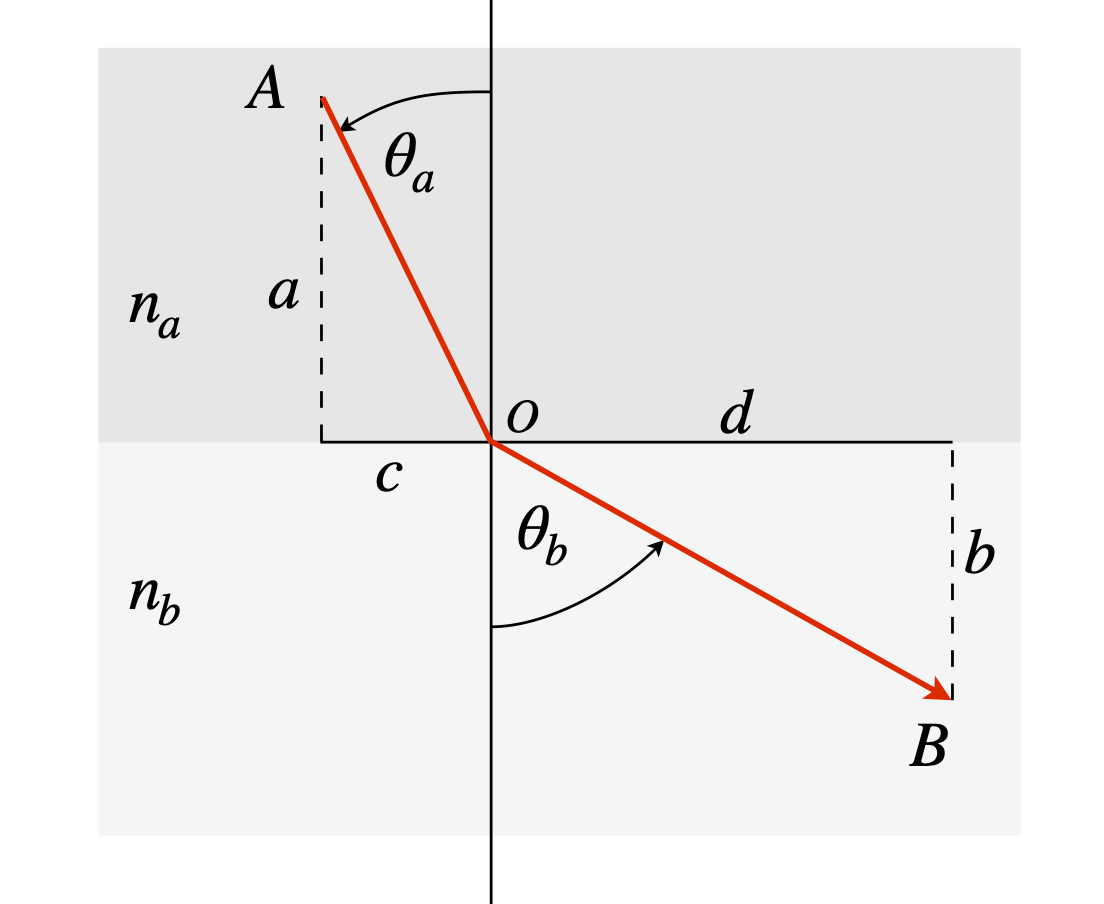# Questions 86 - 92#

Lagrange multiplier questions

## Q86#

Find the shortest distance from the point (2, 2) to the line $$y = e^{-x}$$.

Strategy: To use the Lagrange undetermined multiplier method, first decide what is the constraint and what the function.

## Q87#

Find the shortest distance from the origin to $$y^2 = (4 - x)^3/x$$ , see Fig. 17.

Exercise: Write a python worksheet to calculate the distance from the origin to any curve you choose. Test it on the examples and questions above.

## Q88#

(a) Using the Lagrange multiplier method, maximize the product $$ab$$ with the constraint $$a^2 + b^2 = 1$$.

Strategy: In this example, the variables are $$a$$ and $$b$$, not $$x$$ and $$y$$: change them to $$x$$ and $$y$$ if you find this easier.

## Q89 Photon’s shortest path#

Photons always take a path through a series of optical elements, of any description whatsoever, that minimizes the total time taken. Prove Snell’s law

$\displaystyle n_a \sin(\theta_a) = n_b \sin(\theta_b)$

by calculating the minimum time a photon takes to get from A to B when it crosses a boundary from one medium to another with refractive indices $$n_a$$ and $$n_b$$ and $$n_b \lt n_a$$, as shown in Fig. 32. The speed of light in a medium is $$c/n$$ where $$n$$ is the medium’s refractive index. Use python/SymPy to do the calculation if you wish.Figure 32. Illustrating Snell’s law. The refractive indices $$n_a \gt n_b$$.

Strategy: The constraint is not mentioned, but as A and B are fixed, the constraint must be to make the horizontal distance $$c + d$$ constant. It is not necessary to know this constant because when differentiated it becomes 0. If the vertical distance $$a + b$$ was chosen as the constraint then it is found that $$\lambda$$, the multiplier, disappears from the problem so this cannot be correct.

## Q90 Entropy of mixing#

The entropy of mixing different gases or solutions is $$\displaystyle S=-k_B\sum_{i=1}^n x_i\ln(x_i)$$,

where $$x_i$$ is the mole fraction of component $$i,\; k_B$$ is Boltzmann’s constant, and n the number of species. Show that the maximum entropy of mixing of $$n$$ species is $$S = k_B \ln(n)$$.

Strategy: A thought experiment suggests that the most likely entropy is when each component is present with a mole fraction of 1/$$n$$th of the total if there are $$n$$ species present in the mixture, since this is the most varied way the solution can be divided up into its components.

If there are two components, with mole fraction $$x_1$$ and $$x_2$$ and therefore $$x_2 = 1 - x_1$$, the entropy is

$\displaystyle S = -k_B\big(x_1 \ln(x_1) + (1 - x_1)\ln(1 - x_1)\big)$

The minimum is found by differentiating, which produces

$\displaystyle \frac{dS}{dx_i} = -k_B\big(\ln(x_1) - \ln(1 - x_1)\big) = 0$

which has the solution $$x_1$$ = 1/2 if the sum of the mole fractions add to unity which, by definition, they must. If there were $$n$$ species this approach would become impossible, or at least very tedious, and therefore the Lagrange method is preferable.

## Q91 Maximum entropy distribution#

In the gas phase reaction $$\mathrm{ F + H_2 \to HF_v + H}$$, the product HF is produced in a range of vibrational quantum states, only $$v$$ = 0, 1, 2, 3 being energetically possible under the experimental conditions used. The fraction of HF molecules found in vibrational energy level $$v$$ is $$x_v$$ but these are subject to a constraint such that $$\displaystyle \sum_{n=1}^n x_v = 1$$ where $$n$$ is the total number of levels populated, which happens to be four in this experiment but of course could be different with different reactants.

The distribution of $$x_v$$ vs $$v$$ is expected to maximize the entropy; if this is the case what will the distribution be? This is the same as asking the question ‘what then will be the equation for $$x_i$$’? The vibrational contribution to the entropy (in units of $$k_B$$) is defined as $$\displaystyle S = -\sum_{v=0}n x_v \ln(x_v)$$.

Strategy: First decide what the function to minimize or maximize is, and because there is a constraint use the Lagrange multiplier method. The $$Q$$ function, equation (36), is $$Q = S - \lambda g$$ where $$g$$ is the constraint. The sum of fractional populations, $$x_v$$ is unity and $$S$$ is the entropy function.

## Q92#

Find the maximum and minimum of the function $$f (x, y, z) = 4x + 3y + 10z$$ with the two constraints, $$x+y+z=1$$ and $$x^2 +z^2 =1$$.

Strategy: As there are two constraints there will be two undetermined multipliers; if these are $$\lambda$$ and $$\mu$$ then the $$Q$$ equation is $$Q = f + \lambda g + \mu h$$, where the constraints are the functions $$g$$ and $$h$$ which are the equations in $$x,\; y$$ and $$z$$. Differentiation has also to be performed in $$x,\; y$$ and $$z$$.